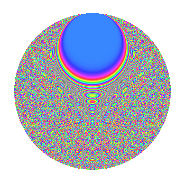# Properties

 Label 3150.2.cqLevel 3150 Weight 2 Character orbit cq Rep. character $$\chi_{3150}(331,\cdot)$$ Character field $$\Q(\zeta_{15})$$ Dimension 1920 Sturm bound 1440

# Related objects

## Defining parameters

 Level: $$N$$ = $$3150 = 2 \cdot 3^{2} \cdot 5^{2} \cdot 7$$ Weight: $$k$$ = $$2$$ Character orbit: $$[\chi]$$ = 3150.cq (of order $$15$$ and degree $$8$$) Character conductor: $$\operatorname{cond}(\chi)$$ = $$1575$$ Character field: $$\Q(\zeta_{15})$$ Sturm bound: $$1440$$

## Dimensions

The following table gives the dimensions of various subspaces of $$M_{2}(3150, [\chi])$$.

Total New Old
Modular forms 5824 1920 3904
Cusp forms 5696 1920 3776
Eisenstein series 128 0 128

## Trace form

 $$1920q + 240q^{4} + O(q^{10})$$ $$1920q + 240q^{4} + 26q^{15} + 240q^{16} + 12q^{17} - 16q^{18} + 12q^{21} + 16q^{23} - 96q^{26} - 48q^{27} - 14q^{30} + 30q^{33} + 14q^{35} - 32q^{39} + 32q^{41} + 28q^{42} - 50q^{45} + 4q^{50} - 12q^{51} - 40q^{53} + 12q^{55} + 96q^{57} + 24q^{58} + 24q^{59} - 2q^{60} + 36q^{62} + 16q^{63} - 480q^{64} + 10q^{65} - 16q^{66} + 96q^{68} + 42q^{69} - 24q^{70} + 36q^{71} + 8q^{72} - 226q^{75} - 112q^{77} - 16q^{78} - 12q^{79} + 52q^{81} + 84q^{83} + 36q^{84} + 88q^{87} + 18q^{89} + 88q^{90} + 12q^{92} - 116q^{93} - 34q^{95} + 48q^{98} + 32q^{99} + O(q^{100})$$

## Decomposition of $$S_{2}^{\mathrm{new}}(3150, [\chi])$$ into newform subspaces

The newforms in this space have not yet been added to the LMFDB.

## Decomposition of $$S_{2}^{\mathrm{old}}(3150, [\chi])$$ into lower level spaces

$$S_{2}^{\mathrm{old}}(3150, [\chi]) \cong$$ $$S_{2}^{\mathrm{new}}(1575, [\chi])$$$$^{\oplus 2}$$

## Hecke Characteristic Polynomials

There are no characteristic polynomials of Hecke operators in the database